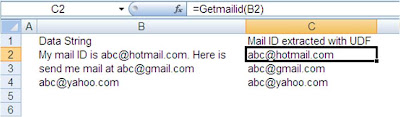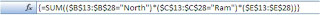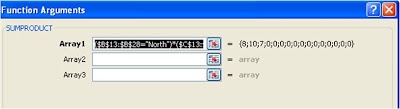## Sunday, November 22, 2009

### Excel Formula : Using Vlookup Function

One Golden Rule while working with Excel Formula's is never enter a hard coded value into Formula. If you can calculate a value with a formula, it should not be hard coded into your formula's

Today we will talk about how this is relevant in using Vlookup Function. We all use Vlookup function in our day to day working with the data in Excel. Given below is the Syntex for Vlookup function just for reference purpose.

VLOOKUP(lookup_value, table_array, col_index_num, [range_lookup])Picture on the above is typical data table used by us. Column C to N are hidden in this just to keep the table visible on screen. We need to get the sales value using vlookup formula. Most of the users (including me till some time back) hardcode col_index_num. Most common use of Vlookup Function for getting data from the table like above will be a formula like this.
=VLOOKUP(B13,B3:P10,15,0)

Here lookup_value is given at Cell B13, table_array is a Range B3:P10, col_index_num is 15 as it is 15th Column starting from Column B, range_lookup is 0 as we are looking for exact match.

In above formula the col_index_num is hard coded. Formula will give correct results till you do not insert or delete columns in between. Once you have done so , all your formulas will not update sales figure but reference some other value.

However with the following formula you can overcome this issue. You can calculate the number of columns with the help of Columns function of Excel.
=VLOOKUP(B13,\$B\$3:\$P\$10,COLUMNS(B3:P3),0)

COLUMNS(B3:P3) will be calculated as 15 and in case you insert any column between table_array your formula will change to VLOOKUP(B13,\$B\$3:\$Q\$10,COLUMNS(B3:Q3),0). Now columns function withing your formula will be calculated as 16 giving you correct value. This makes your Vlookup function dynamic.

Do refer to my earlier tip on Dynamic Range Names to make table_array also dynamic.

You are welcome to share your way of entering Vlookup function by way of comments to this post.

## Wednesday, November 18, 2009

### Excel Macro : Email Address Extract from String

Last week I got a mail from my friend asking for help in extracting email IDs from data available with him. The position of the mail IDs within the text string was not same and he was finding it difficult to us extract email addresses.

I wrote a quick UDF for him which did the job. I am sharing same with you as you may find it use full.

Sample of the data and mail IDs extracted with the UDF is as belowHere is the VBA code to Email Address Extract from String. You will need to copy this code to regular VBA module of your workbook
 `Function Getmailid(cell As Range) As StringDim Textstrng As StringTextstrng = cell.TextPosition@ = InStr(1, Textstrng, "@")EmStart = InStrRev(Textstrng, " ", Position@)If EmStart = 0 Then EmStart = 1EmEnd = InStr(Position@, Textstrng, " ")If EmEnd = 0 Then EmEnd = Len(Textstrng) + 1mailid = Trim(Mid(Textstrng, EmStart, EmEnd - EmStart))If Right(mailid, 1) = "." ThenGetmailid = Left(mailid, Len(mailid) - 1)ElseGetmailid = mailidEnd IfEnd Function`

## Friday, November 13, 2009

### Excel Macro : Hide Cell Content from Printing

Some times we have some information in worksheet which is we need to input but do not want that information to be printed. With the following macro code you can achieve the desired results

You will need to copy this code to workbook module of your workbook

 `Private Sub Workbook_BeforePrint(Cancel As Boolean) Application.EnableEvents = False Cancel = True A = Range("A1").NumberFormat Range("A1").NumberFormat = ";;;" Application.Dialogs(xlDialogPrint).Show Range("A1").NumberFormat = A Application.EnableEvents = TrueEnd Sub`

As per above code the contents of Range A1 will not be printed, you will need to change this reference to your cell.

.

## Friday, November 6, 2009

### Excel Formula : Sumif Multiple Criteria

This is in continuation of my earlier post on Countif Multiple Criteria The same logic can be extended to calculate sum based on multiple criteria.

In case you have Excel 2007, you can use built in function Sumifs . This will let you add multiple conditions.In case of Excel 2003 or earlier version using Pivot table will get you the results easily but if You don't have liberty to use Pivot, then you will need formula trick. Consider that you have a data table like this and you need to know what is sales in "North" region by sales man "Ram".

Following sumproduct formula will do the calculation for you.

=SUMPRODUCT((\$B\$13:\$B\$28="North")*(\$C\$13:\$C\$28="Ram")*(\$E\$13:\$E\$28))

Another way is to write an array formula.
=SUM((\$B\$13:\$B\$28="North")*(\$C\$13:\$C\$28="Ram")*(\$E\$13:\$E\$28))
However this will need to be confirmed with CTRL+Shift+Enter (CSE)

After CSE formula will show {} in formula bar. This will covert it to an array formula. The formula will look like following picture after CSE in formula barI suggest you to use Sumproduct formula as you may find it difficult to enter array formula.

If you see the dialog for sumproduct formula entry you will understand the reasons behind this recommendation. Actually sumproduct is a workaround to enter array formula.If you see above dialog screen , you will notice

1. When you enter ranges, you are actually entering array's.
2. This formula is entered in single array only to get the results.

If you try to use sumproduct as normal formula to calculate sum based on multiple conditions , you will not get the results.

Actually multiple condistional sum can be calculated using arrays only and sumproduct allows you to enter array's easily and you can over come the difficulty of entering array formula.